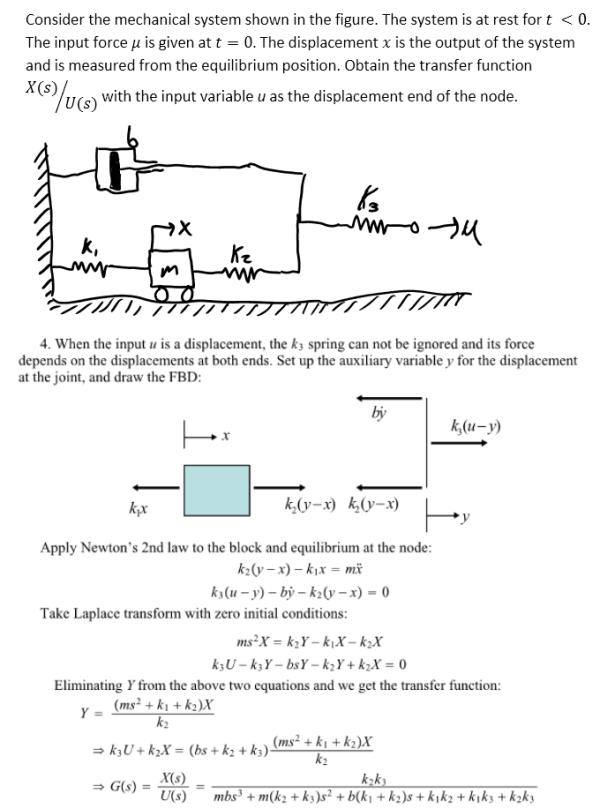Consider the mechanical system shown in the figure. The system is at rest for t greater than 0. The input force u is given at t=0. The displacement x is the output of the system and is measured from the equilibrium position. Obtain the transfer function X(s)/U(s) with the input variable u as the displacement end of the node.Consider the mechanical system shown in the figure. The system is at rest for t greater than 0. The input force u is given at t=0. The displacement x is the output of the system and is measured from the equilibrium position. Obtain the transfer function X(s)/U(s) with the input variable u as the displacement end of the node.

System Dynamics Page 2 dynamics dynamics dynamics dynamics dynamics dynamics dynamics System dynamics Page 3